Post 1: Simple Sine and Cosine Wave Generation

Objective

To show the most basic features and development of my python module:

• Generate Sin and Cosine objects, with all user defined parameters
• Perform FFT on object waves thorught the use of method functions
• Prduce standalone plots, subplots, and group-plots

Background: What is the point?

I graduated with a degree in Electrical Engineering with a concerntration in digital signal processing, communications, and linear systems. But more importantly, those concentrations were my actual true interests. The mathematics drew me towards them but the practially and implications of such topics kept me.

Since graduation, just over a year a from when I am writing this, my job has not called on me to use any of these topics. The job I have is in electrical engineering and it is a fantastic place to work, however I realized that I could get stuck. If I am going to be an engineer I want to be working in the realm it is most easy to apply myself. As I thought about this and asked myself some questions about my future, I realized I needed to get back to my innate interests.

After deciding to get back to my roots I found myself needing to 'catch up'. I spent alot of time on my computer reading, studying, implementing, and coding various topics. However, with that, I noticed that much of the process in implementing ideas into the python code, was syntactual - if that is even a word - and less time and thought energy was being poured into the topics themselves. Furthermore, development would vary from python script to python script. That was becasue python allows users a million differnet ways to do the same thing. My scripts, from topic to topic were lacking true standardization and that is want I wanted.

This caused me to look if a python module existed that emulated a signal generator, oscilloscope, and spectrum analyzer. I didnt really find exaclty what I was looking for. It is entirely possible that I didnt look hard enough. But as I was looking I thought to myself that, "Even if I find the module, I know that I will always wonder how the back end works? And what if they dont have a feature that I want/need? What if it doesnt work exactly how I see it in my head?" That when I just decided that I will write a python module for myself.

So as a result I began writing it. That is what these posts will be show casing: how to work with my module (which I hope to put on a git hub for the world to use and improve) and the topics that like to work on, in hopes that it could be educational for some.

As I write this the module is in its beginning stages. There are many features, checks, and bugs that need to be sorted but that is merely a matter of time and energy.

Code

As with any python script, the user needs to import necessary modules. Most of my posts will always try to only import my module (which is defintely up for a name change). My module actually import a couple python modules. It makes great use of numpy, matplotlib, and scipy. But again, I will strongly refrain from importing in the notebooks becasue I really want to put stress on the module to contain all the features I need to do the work.

In :
""" Imports"""
%matplotlib inline
import sys
sys.path.append('C:\Users\blah\Documents\Python Scripts\Scripts\Signal_Consruction')
import sigCon as sc

Step up givens
In :
ac = int(7)
dc = int(0)
per = str("20 ms")
ft = str("50 kHz")
fs = str("2250 kHz")

As with any signal generation, the parameters of the signals need to be define. That is unescapeable.

Generate Signal: Example sine and cosine wave
In :
osin = sc.sin(AC = ac, DC = dc, FT = ft, FS = fs, N = 225)
ocos = sc.cos(AC = ac, DC = dc, FT = ft, FS = fs, N = 225)

This is a simple creation of a signal object. My intention was to treat a signal more like an object than then simply a numpy array of values. To me, it is more intuitive to structure the signals as such.

Moreover, structuring the signlas as objects allows me to build member functions into the into the signals.

Notice that argument calls for the constructor implement all capitalized key words. That is meant to be a consisent theme throughout the module.

• AC:magnitude of the Analog Component of the Signal
• DC:magnitude of the Direct Component of the Signal
• PER:Period of the Test Signal
• FT:Frequency of Test Signal
• FS:Sampling Frequency of the Test Signal
• N:Number of samples
Plotting: Standard new figure plot
In :
osin.plot('line', XLABEL = "Sine Wave")
ocos.plot('stem', COLOR = 'k', XLABEL = "Cosine Wave" )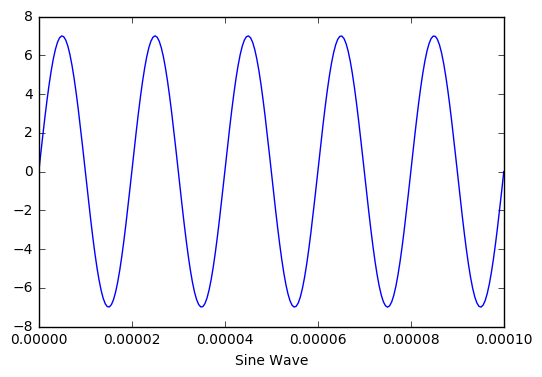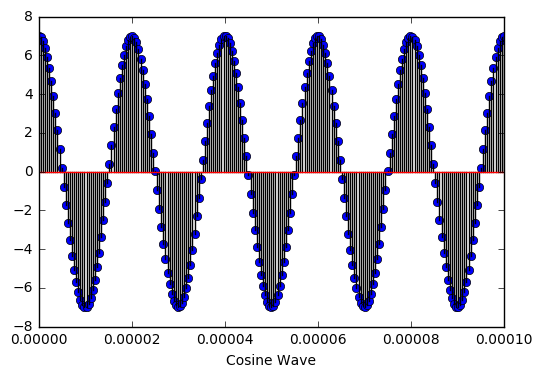Plotting: Subplots
In :
osin.splot(211, DOMAIN = 'time', COLOR = "k")
osin.fft()
osin.splot(212, DOMAIN ='f', TYPE = 'stem', COLOR = 'k', AXIS = 'x,0, 20')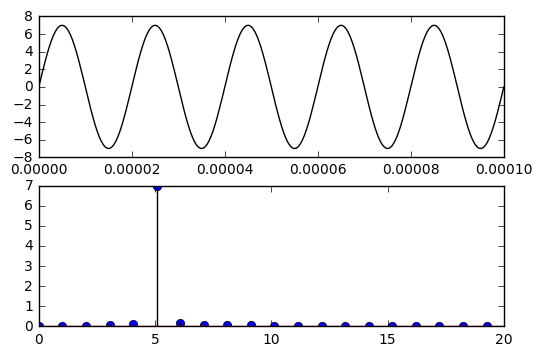In :
ocos.splot(211, COLOR = "k", YLABEL = 'Time Domain', TITLE = "Cosine Wave")
ocos.fft()
ocos.splot(212, DOMAIN ='f', TYPE = 'stem', COLOR = 'k', YLABEL = 'Freq. Domain', AXIS = "x, 0, 20")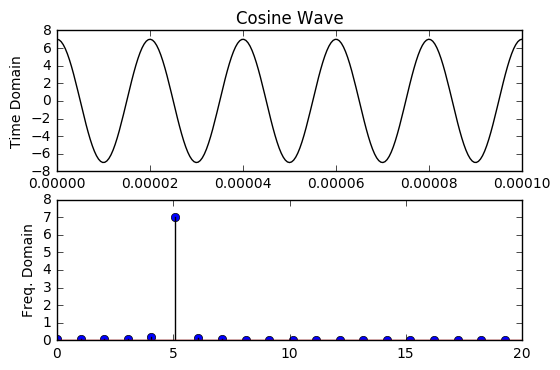Plotting: Group plots (multiple plots on single figure)
In :
osin.gplot(TITLE = "Group Plot: Sine and Cosine")
ocos.gplot()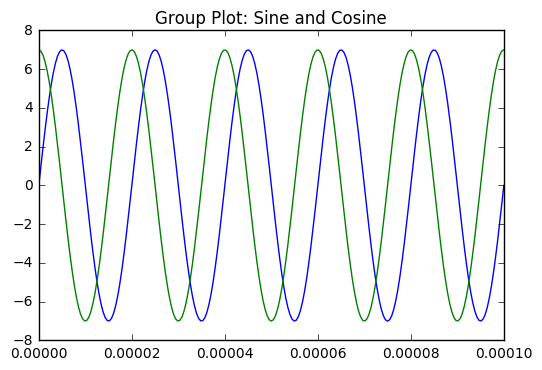In [ ]: